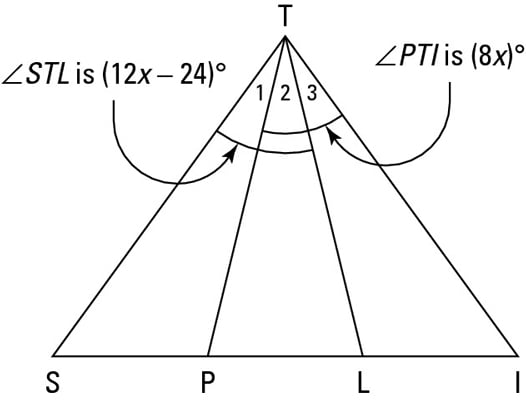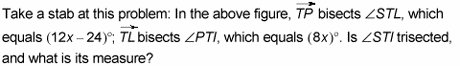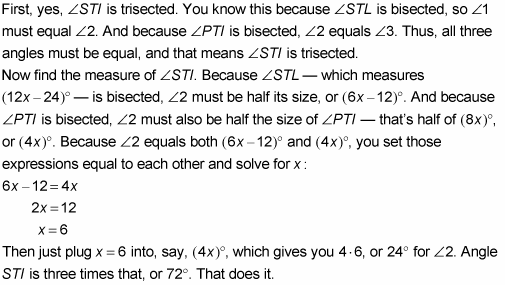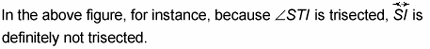##### Geometry Workbook For DummiesThe terms angle bisection and angle trisection describe two ways in which you can divide up an angle equally into two (or three) smaller, congruent angles. Their definitions are often used in proofs.

• Angle bisection: A ray that cuts an angle into two congruent angles bisects the angle. The ray is called the angle bisector.

• Angle trisection: Two rays that divide an angle into three congruent angles trisect the angle. These rays are called angle trisectors.Nothing to it.When rays trisect an angle of a triangle, the opposite side of the triangle is never trisected by these rays.